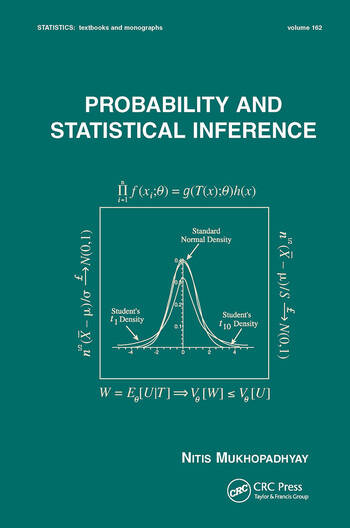# Probability and Statistical Inference

## 1st Edition

CRC Press
Published March 22, 2000
Textbook - 665 Pages
ISBN 9780824703790 - CAT# DK1646
Series: Statistics: A Series of Textbooks and Monographs

For Instructors Request Inspection Copy

was \$140.00

USD\$112.00

SAVE ~\$28.00

FREE Standard Shipping!

## Preview

### Summary

Priced very competitively compared with other textbooks at this level!
This gracefully organized textbook reveals the rigorous theory of probability and statistical inference in the style of a tutorial, using worked examples, exercises, numerous figures and tables, and computer simulations to develop and illustrate concepts.

Beginning with an introduction to the basic ideas and techniques in probability theory and progressing to more rigorous topics, Probability and Statistical Inference
• studies the Helmert transformation for normal distributions and the waiting time between failures for exponential distributions
• develops notions of convergence in probability and distribution
• spotlights the central limit theorem (CLT) for the sample variance
• introduces sampling distributions and the Cornish-Fisher expansions
• concentrates on the fundamentals of sufficiency, information, completeness, and ancillarity
• explains Basu's Theorem as well as location, scale, and location-scale families of distributions
• covers moment estimators, maximum likelihood estimators (MLE), Rao-Blackwellization, and the Cramér-Rao inequality
• discusses uniformly minimum variance unbiased estimators (UMVUE) and Lehmann-Scheffé Theorems
• focuses on the Neyman-Pearson theory of most powerful (MP) and uniformly most powerful (UMP) tests of hypotheses, as well as confidence intervals
• includes the likelihood ratio (LR) tests for the mean, variance, and correlation coefficient
• summarizes Bayesian methods
• describes the monotone likelihood ratio (MLR) property
• handles variance stabilizing transformations
• provides a historical context for statistics and statistical discoveries
• showcases great statisticians through biographical notes

Employing over 1400 equations to reinforce its subject matter, Probability and Statistical Inference is a groundbreaking text for first-year graduate and upper-level undergraduate courses in probability and statistical inference who have completed a calculus prerequisite, as well as a supplemental text for classes in Advanced Statistical Inference or Decision Theory.
• #### Instructors

We provide complimentary e-inspection copies of primary textbooks to instructors considering our books for course adoption.